# 2023年成考高起点每日一练《数学(文史)》11月20日

## 试卷预览

• 1. 设集合M={x||x-2||＜2}，N={0,1,2,3,4}，则M∩N=（）

A{2}

B{0,1,2}

C{1,2,3}

D{0,1,2,3,4}

• 2. 在△ABC中，三边为a、b、c，∠B=60°，则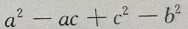的值是（）

A大于零

B小于零

C等于零

D不能确定

• 3. 命题甲:x>y且xy>0,命题乙：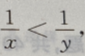则（）

A甲是乙的充分条件,但不是必要条件

B甲是乙的必要条件，但不是充分条件

C甲是乙的充分必要条件

D甲不是乙的必要条件也不是乙的充分条件

• 4. 已知点M(-2,5),N(4,2),点P在上，且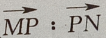=1:2，则点P的坐标为（）

A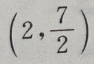B(0,4)

C(8,2)

D(2,1)

• 1. 在△ABC中，AB=2，BC=3,B=60°，求AC及△ABC的面积
• 2. 设函数f(x)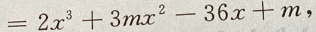且f'(-1)=-36 (Ⅰ)求m (Ⅱ)求f(x)的单调区间
• 3. 设函数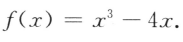(I）求f'(2)；
(II）求f(x）在区间[一1,2]的最大值与最小值．
• 4. 如图：已知在△ADC中，∠C=90°，∠D=30°，∠ABC=45°，BD=20，求AC(用小数表示，保留一位小数)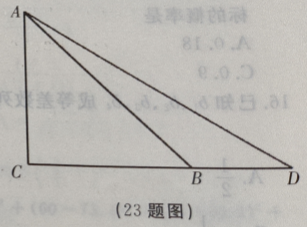• 1. 已知向量a=(3,2),b=(-4,x),且a⊥b，则x=（）
• 2. 任选一个不大于20的正整数,它恰好是3的整数倍的概率是（）